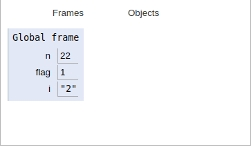# Python Program for Check if all digits of a number divide it

In this article, we will learn about the solution and approach to solve the given problem statement.

Problem statement −Given a number n, find whether all digits of n divide it or not.

Here we will be checking that there is no 0 in the given number because this will give divide by zero exception and hence we must return no as an answer

Otherwise, we must check whether all digits are able to divide the number by using a temporary variable flag which allows declaring a check condition.

Now let’s have a look at the implementation −

## Example

n=int(input())
flag=1
for i in str(n):
if int(i)!=0 and n%int(i)==0:
flag=1
else:
flag=0
if(flag==1):
print("Yes")
else:
print("No")

## Output

Yes(22)

All the variables are declared in global scope as shown in the image below## Conclusion

In this article, we learnt about the approach to Check if all digits of a number divide it.